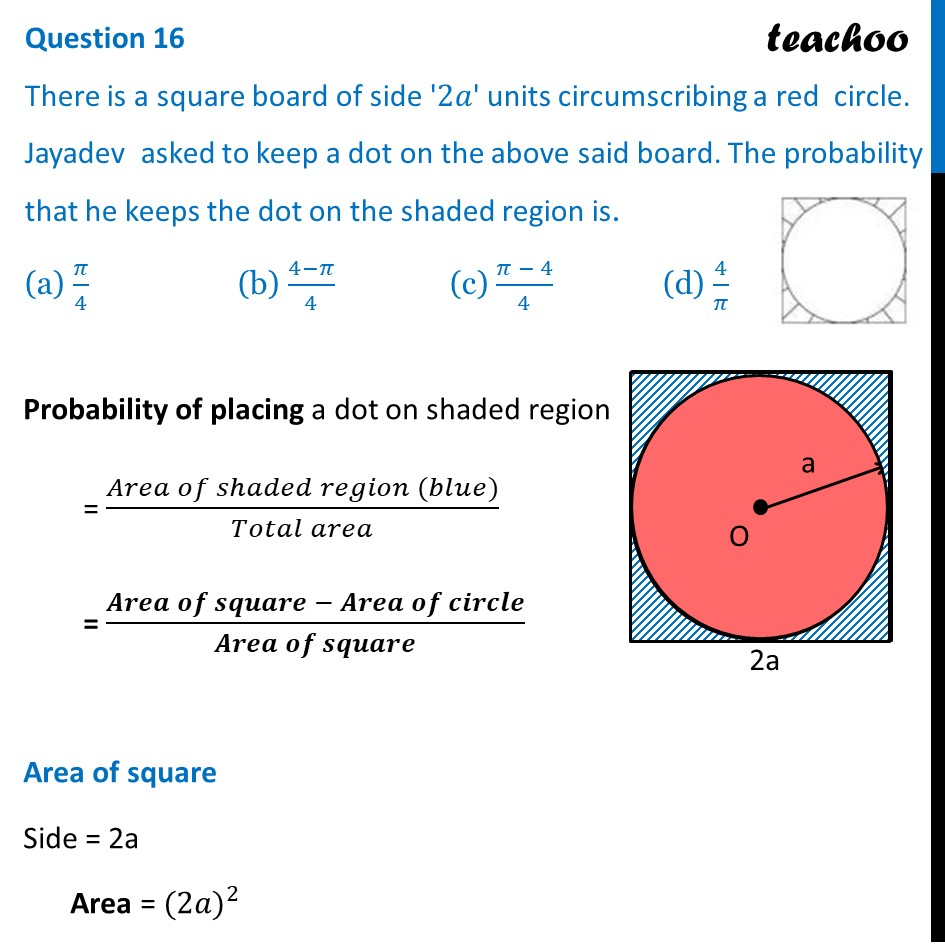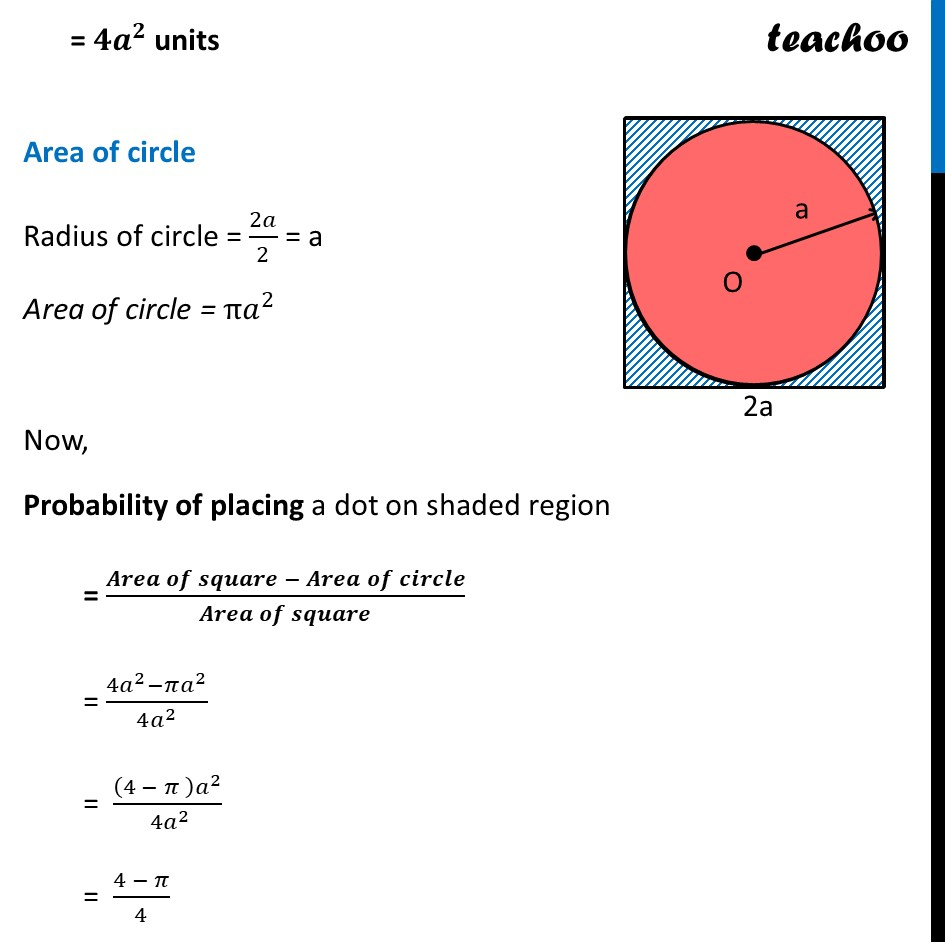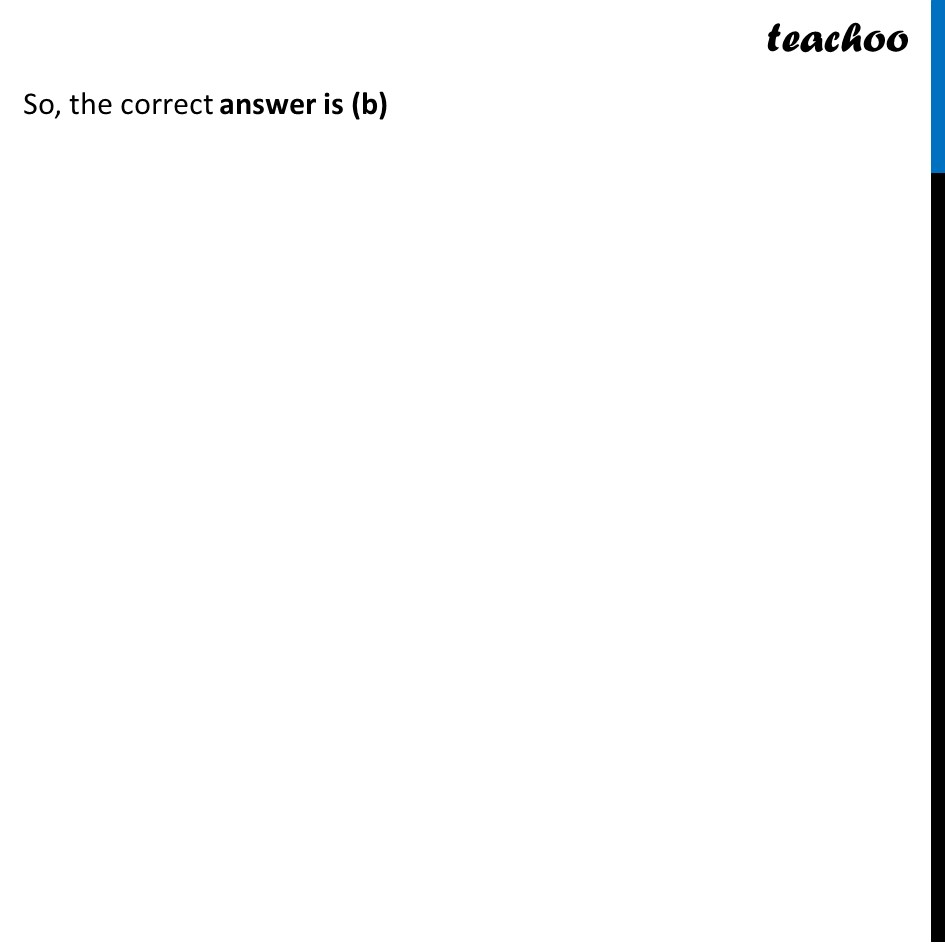CBSE Class 10 Sample Paper for 2024 Boards - Maths Standard

Class 10
Solutions of Sample Papers for Class 10 Boards

## (a) π/4   (b) (4-π)/4   (c) (π - 4)/4    (d) 4/πLearn in your speed, with individual attention - Teachoo Maths 1-on-1 Class

### Transcript

Probability of placing a dot on shaded region = (𝐴𝑟𝑒𝑎 𝑜𝑓 𝑠ℎ𝑎𝑑𝑒𝑑 𝑟𝑒𝑔𝑖𝑜𝑛 (𝑏𝑙𝑢𝑒))/(𝑇𝑜𝑡𝑎𝑙 𝑎𝑟𝑒𝑎) = (𝑨𝒓𝒆𝒂 𝒐𝒇 𝒔𝒒𝒖𝒂𝒓𝒆 − 𝑨𝒓𝒆𝒂 𝒐𝒇 𝒄𝒊𝒓𝒄𝒍𝒆)/(𝑨𝒓𝒆𝒂 𝒐𝒇 𝒄𝒊𝒓𝒄𝒍𝒆) Area of square Side = 2a Area = 〖(2𝑎)〗^2 =〖 𝟒𝒂〗^𝟐 units Area of circle Radius of circle = 2𝑎/2 = a Area of circle = π𝑎^2 Now, Probability of placing a dot on shaded region = (𝑨𝒓𝒆𝒂 𝒐𝒇 𝒔𝒒𝒖𝒂𝒓𝒆 − 𝑨𝒓𝒆𝒂 𝒐𝒇 𝒄𝒊𝒓𝒄𝒍𝒆)/(𝑨𝒓𝒆𝒂 𝒐𝒇 𝒄𝒊𝒓𝒄𝒍𝒆) = (4𝑎^2−𝜋𝑎^2)/(4𝑎^2 ) = ((4 − 𝜋 ) 𝑎^2)/〖 4𝑎〗^2 = (4 − 𝜋)/4So, the correct answer is (b)